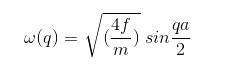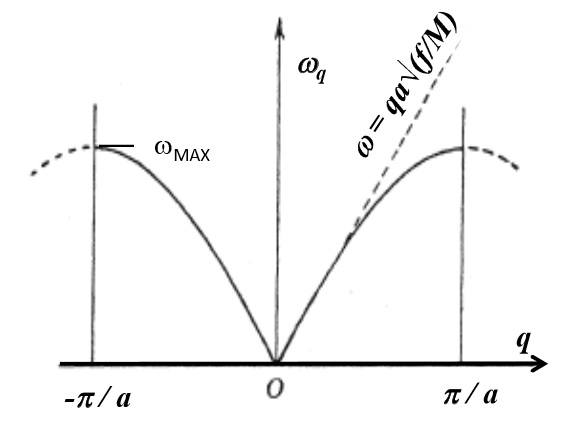# The plot of a linear relation given an equation

rwooduk

## Homework Statement

$$\omega (q)= \sqrt{( \frac{4f}{m})} sin\frac{qa}{2}$$N/A

## The Attempt at a Solution

I dont understand the linear line given on the graph. For low q (or as q tends to zero) it says the relationship is linear. But as q tends to zero for the given equation I dont see how the equation goes to:

##\omega (q)= qa \sqrt{\frac{f}{m}}##

please could someone explain this, it's not a homework question, just going through the notes we have been given.

thanks in advance for any help

Last edited by a moderator:

Homework Helper
When the argument of the function sin(x) is small, x<<1, sin(x)≈x. So ##ω(q)=\sqrt{\frac{4f}{m}} \sin(\frac{qa}{2}) = \sqrt{\frac{4f}{m}} \frac{qa}{2}##.
Pull out 4 from the square root, ##ω(q)=2\sqrt{\frac{f}{m}} \frac{qa}{2}##, simplify by 2.

•rwooduk
rwooduk
When the argument of the function sin(x) is small, x<<1, sin(x)≈x. So ##ω(q)=\sqrt{\frac{4f}{m}} \sin(\frac{qa}{2}) = \sqrt{\frac{4f}{m}} \frac{qa}{2}##.
Pull out 4 from the square root, ##ω(q)=2\sqrt{\frac{f}{m}} \frac{qa}{2}##, simplify by 2.

cant believe i couldnt see that! thanks very much for your help!